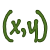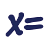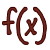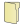Games
Problems
Go Pro!

# Algebra

Pro Problems > Math > Algebra

# Featured Pro ProblemsFind the sum of x and y, if the following quadratic equations in x and y are true.Two people go to the same store and make the same purchases, but one of them is treated unfairly in the pricingRational expressions word problemThe sum of two numbers is six more than twice the smaller number, and if the larger number is multiplied by three, the result is ten less than seven...A problem involving the number of words in seven books on a shelf - arithmetic sequencesA hypothetical problem in which the treasury introduces a new coin into circulationTwo time-based arithmetic series combined at different ratesDetermine how many floors a skyscraper has, based on information about someone climbing the stairs# Blogs on This SiteReviews and book lists - books we love!The site administrator fields questions from visitors.Like us on Facebook to get updates about new resources